# Motion in one Dimension

#### Comparative Study of Instantaneous Speed and Instantaneous Velocity

Comparative Study of Instantaneous Speed and Instantaneous Velocity
Instantaneous velocity or simply velocity is defined as rate of change of particle's position with time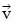=where the position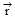of a particle at any instant changes by Δin a small time Δ t. he magnitude of velocity is called speed i.e. speed = | velocity | i.e. v = ||
Note : In straight line motion there is no change in direction soand v both have same meaning.
Note :
(a) Velocity is a vector while speed is a scalar having same units (m/s) and dimension [LT–1]
(b) If during motion velocity remains constant throughout a given interval of time, the motion is said to be uniform and for uniform motion,= constant =However converse may or may not be true i.e. If=, the motion may or may not be uniform.
(c) If velocity is constant, speed ( = | velocity |) will also be constant. However conversdree may or may not be true i.e. if speed = constant, velocity may or may not be constant as velocity has a direction in addition to magnitude which may or may not change. e.g. in case of uniform rectilinear motion.= constant and so speed || = constant while in case of uniform circular motion, v = constant but≠ constant due to change in direction.
(d) Velocity can be positive or negative, as it is a vector but speed can never be negative as it is the magnitude of velocity i.e. v = ||
(e) If displacement is given as a function of time, the time derivative of displacement will give velocity and modulus of velocity gives speed.
e.g. s = A0 – A1t + A2t2, v =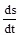= – A1 + 2A2t. So, initially (t = 0), velocity = – A1, while speed = |–A1| = A1
Special Note : It is common misconception, that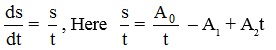Which is totally different from the above value of.
(f) As by definition, v =, the slope of displacement versus time graph gives velocity.i.e. v == tan θ = slope of s-t curve
(g) As, v =⇒ ds = vdt
From figure vdt = dA. so, dA = ds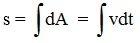Area under velocity versus time graph with proper algebraic sign gives displacement while without sign gives distance.e.g. From the adjoining v-t graph.
The distance travelled by body in time t3 = Area I + Area II + Area III and the displacement of body = Area II – Area III – Area I

If you want to give information about online courses to other students, then share it with more and more on Facebook, Twitter, Google Plus. The more the shares will be, the more students will benefit. The share buttons are given below for your convenience.

#### IIT (Class X)

• Unit, Dimension & Error
• Vectors
• Motion in One Dimension
• PROJECTILE MOTION
• NEWTON'S LAWS OF MOTION & FRICTION
• WORK, POWER, ENERGY & CONSERVATION LAWS
• CIRCULAR MOTION & ROTATIONAL DYNAMICS
• GRAVITATION